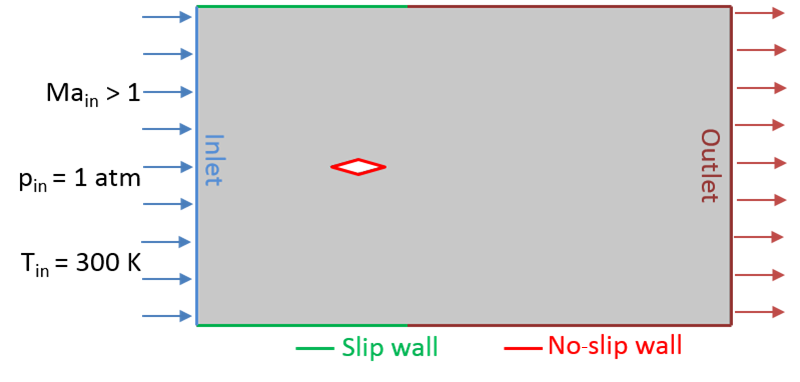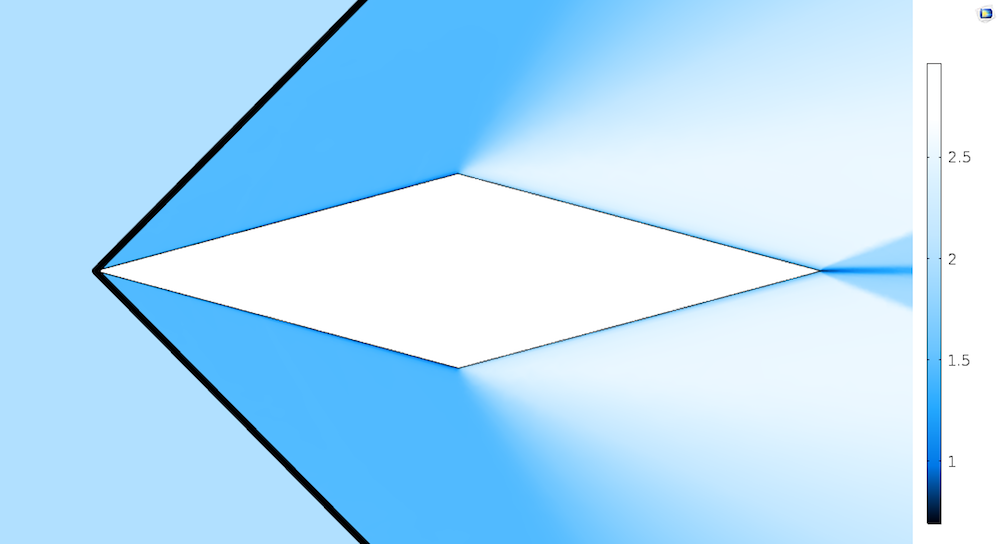# 如何在 COMSOL Multiphysics® 中模拟超音速流动

2017年 8月 10日

### 亚音速、音速和超音速流动之间有什么区别？### 计算激波的激波角和马赫数

\tan\delta = 2 \cot\sigma \left[ Ma_{0}^2 \sin^2\sigma -1 \over {Ma_{0}^2 (\gamma + \cos 2\sigma) + 2}\right]

Ma_1 = \sqrt{(\gamma+1)^2 Ma_0^4 \sin^2\sigma-4(Ma_0^2 \sin^2\sigma -1)(\gamma Ma_0^2 sin^2\sigma +1) \over [2\gamma Ma_0^2 \sin^2\sigma – (\gamma-1)][(\gamma -1)Ma_0^2 \sin^2 \sigma + 2]}

\theta = \sqrt{\gamma +1 \over \gamma -1} \left[ \tan^{-1} \left( \sqrt{{\gamma -1 \over \gamma + 1}(Ma_2^2 -1)}\right) – \tan^{-1} \left( \sqrt{{\gamma -1 \over \gamma + 1}(Ma_1^2 -1)}\right)\right]- \left[\tan^{-1}\left(\sqrt{Ma_2^2-1}\right) – \tan^{-1}\left(\sqrt{Ma_1^2-1}\right) \right]

### 在 COMSOL Multiphysics® 中模拟通过菱形翼型的超音速流动0 2.0 2.0 1.0 1.0 300 300
1 1.45 1.44 2.22 2.21 378 381
2 2.55 2.53 0.40 0.41 231 237### 更多资源

#### 评论 (4)

##### 留言##### 春龙 海
2022-07-15

Hi,Tholeti, can you send me this model you describe here？ thank you, wait for your reply##### Siva Sashank Tholeti
2022-07-15 COMSOL 员工

Hi,##### xiaoling chen
2023-03-20##### 越 赵
2023-03-22 COMSOL 员工